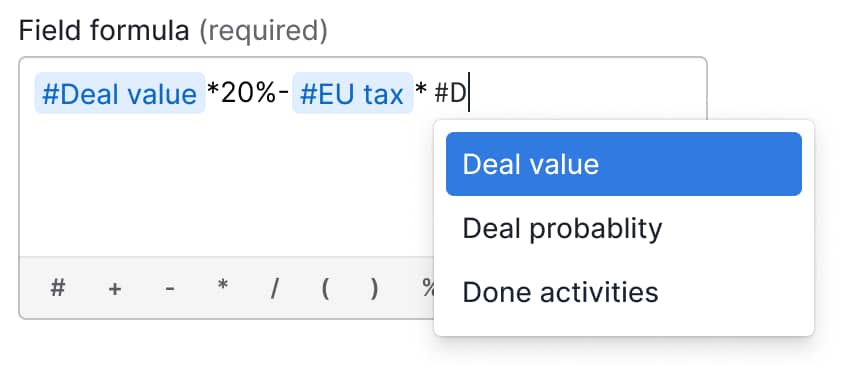🚗  Business experts on board! Tune in to episode one of The Pipedrive to get inspired. Watch now.

English (US)
Deutsch
Español (América Latina)
Français
Português (BR)
Čeština
Eesti
Español (España)
Bahasa Indonesia
Italiano
Japanese (日本語)
Korean (한국어)
Latviešu valoda
Nederlands
Norsk
Polski
Русский
Suomi
Svenska
Türkçe
Українська
Chinese (繁體中文)

# Calculate formulas with the new custom field option in deals###### Topics
How do I set up a formula in a custom field?
How does the formula function work?

Pipedrive users on Professional, Power and Enterprise plans can now create formula fields in deals that calculate important totals and metrics automatically, based on data in other fields.

We have added a new custom field option to Pipedrive, which enables users to calculate results based on the data that has been inputted into other fields. Once the formula has been set up, any time a deal is added or edited it will calculate the relevant total automatically – as long as the other fields in the formula are present in the deal.

The new formulas have many applications, but here are a few ways you things you could calculate:

• Commissions for your sales reps based on a percentage of every deal value in your pipeline

• The amount a customer is yet to pay on a deal if they are paying in installments

• The total value of a subscription based on the customer’s monthly bills

## How do I set up a formula in a custom field?

Setting up a formula is simple:

1. When creating or editing a deal field, turn on the new “Apply formula to deals” switch, which will make the formula textbox and apply options appear

2. Using the hashtag (“#”), enter the numeric and/or monetary fields that will be used in the calculation and Pipedrive will suggest fields based on what you type in – at least one has to be added to make the formula work

3. Use mathematical symbols to complete the formulaHere are the mathematical symbols you can add:

• Plus (“+”), minus (“-”), multiply (“*”) and divide (“/”) all work as ordinary math symbols – at least one symbol must be included for the formula to work

• Brackets, “(“ and “)” group sections of the equation together

• The percentage symbol “%” allows you to subtract or add a share of an input or number

• The numbers 0 to 9 and the decimal point “.”## How does the formula function work?

When you’ve completed your formula, you can choose whether it is calculated for all your open and new deals or just new ones you create.

A pop-up notification will appear, showing you the progress of the formula calculation. Formula values are then automatically calculated and updated when:

• Formulas are created/edited

• Deals are created

• Deal fields included in the formula are edited

• You recalculate a formula (in the deal detail or list view, or when bulk editing deals)

If the formula you created doesn’t have the correct structure, the “Field formula” box will highlight in red and an explanation message will appear.

The formula custom field option is being rolled out in February to Professional, Power and Enterprise customers. If you would like to try Pipedrive, you can sign up to a free trial for 14 days.

### Share your thoughts with our Community

Start or continue the conversation with like-minded sales and marketing professionals on our Community.